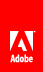Date and time example: Simple analog clock

Flash Player 9 and later, Adobe AIR 1.0 and later

A simple analog clock example illustrates these two date and time concepts:

• Getting the current date and time and extracting values for the hours, minutes, and seconds

• Using a Timer to set the pace of an application

To get the application files for this sample, see www.adobe.com/go/learn_programmingAS3samples_flash . The SimpleClock application files can be found in the folder Samples/SimpleClock. The application consists of the following files:

File

Description

SimpleClockApp.mxml

or

SimpleClockApp.fla

The main application file in Flash (FLA) or Flex (MXML).

com/example/programmingas3/simpleclock/SimpleClock.as

The main application file.

com/example/programmingas3/simpleclock/AnalogClockFace.as

Draws a round clock face and hour, minute, and seconds hands based on the time.

Defining the SimpleClock class

The clock example is simple, but it’s a good idea to organize even simple applications well so you could easily expand them in the future. To that end, the SimpleClock application uses the SimpleClock class to handle the startup and time-keeping tasks, and then uses another class named AnalogClockFace to actually display the time.

Here is the code that defines and initializes the SimpleClock class (note that in the Flash version, SimpleClock extends the Sprite class instead):

public class SimpleClock extends UIComponent
{
/**
* The time display component.
*/
private var face:AnalogClockFace;

/**
* The Timer that acts like a heartbeat for the application.
*/
private var ticker:Timer;

The class has two important properties:

• The face property, which is an instance of the AnalogClockFace class

• The ticker property, which is an instance of the Timer class

The SimpleClock class uses a default constructor. The initClock() method takes care of the real setup work, creating the clock face and starting the Timer instance ticking.

Creating the clock face

The next lines in the SimpleClock code create the clock face that is used to display the time:

/**
* Sets up a SimpleClock instance.
*/
public function initClock(faceSize:Number = 200)
{
// creates the clock face and adds it to the display list
face = new AnalogClockFace(Math.max(20, faceSize));
face.init();

// draws the initial clock display
face.draw();

The size of the face can be passed in to the initClock() method. If no faceSize value is passed, a default size of 200 pixels is used.

Next, the application initializes the face and then adds it to the display list using the addChild() method inherited from the DisplayObjectContainer class. Then it calls the AnalogClockFace.draw() method to display the clock face once, showing the current time.

Starting the timer

After creating the clock face, the initClock() method sets up a timer:

// creates a Timer that fires an event once per second
ticker = new Timer(1000);

// designates the onTick() method to handle Timer events

// starts the clock ticking
ticker.start();

First this method instantiates a Timer instance that will dispatch an event once per second (every 1000 milliseconds). Since no second repeatCount parameter is passed to the Timer() constructor, the Timer will keep repeating indefinitely.

The SimpleClock.onTick() method will execute once per second when the timer event is received:

public function onTick(event:TimerEvent):void
{
face.draw();
}

The AnalogClockFace.draw() method simply draws the clock face and hands.

Displaying the current time

Most of the code in the AnalogClockFace class involves setting up the clock face’s display elements. When the AnalogClockFace is initialized, it draws a circular outline, places a numeric text label at each hour mark, and then creates three Shape objects, one each for the hour hand, the minute hand, and the second hand on the clock.

Once the SimpleClock application is running, it calls the AnalogClockFace.draw() method each second, as follows:

/**
* Called by the parent container when the display is being drawn.
*/
public override function draw():void
{
// stores the current date and time in an instance variable
currentTime = new Date();
showTime(currentTime);
}

This method saves the current time in a variable, so the time can’t change in the middle of drawing the clock hands. Then it calls the showTime() method to display the hands, as the following shows:

/**
* Displays the given Date/Time in that good old analog clock style.
*/
public function showTime(time:Date):void
{
// gets the time values
var seconds:uint = time.getSeconds();
var minutes:uint = time.getMinutes();
var hours:uint = time.getHours();

// multiplies by 6 to get degrees
this.secondHand.rotation = 180 + (seconds * 6);
this.minuteHand.rotation = 180 + (minutes * 6);

// Multiply by 30 to get basic degrees, then
// add up to 29.5 degrees (59 * 0.5)
// to account for the minutes.
this.hourHand.rotation = 180 + (hours * 30) + (minutes * 0.5);
}

First, this method extracts the values for the hours, minutes, and seconds of the current time. Then it uses these values to calculate the angle for each hand. Since the second hand makes a full rotation in 60 seconds, it rotates 6 degrees each second (360/60). The minute hand rotates the same amount each minute.

The hour hand updates every minute, too, so it can show some progress as the minutes tick by. It rotates 30 degrees each hour (360/12), but it also rotates half a degree each minute (30 degrees divided by 60 minutes).// Ethnio survey code removed